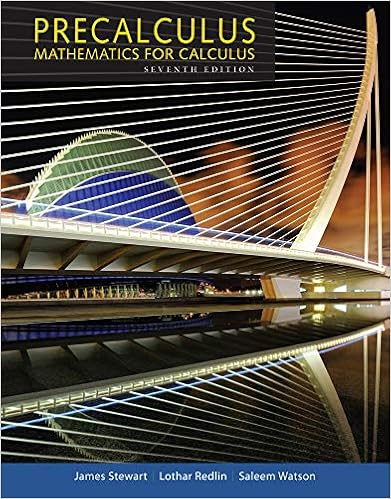# 2 s t t s theorem let s r 2 r 2 and t r 2 r 2 be

• Notes
• 58
• 100% (1) 1 out of 1 people found this document helpful

This preview shows page 44 - 48 out of 58 pages.

##### We have textbook solutions for you!
The document you are viewing contains questions related to this textbook.The document you are viewing contains questions related to this textbook.
Chapter 10 / Exercise 12
Precalculus: Mathematics for Calculus
Redlin/StewartExpert Verified
(2) S T T S . Theorem. Let S : R 2 R 2 and T : R 2 R 2 be matrix transformations with matrices A and B respec- tively. Then S T is the matrix transformation with matrix AB . Proof. We have S(v) = Av and T(v) = Bv . Hence [S T](v) = S(T(v)) = S(Bv) = ABv.
##### We have textbook solutions for you!
The document you are viewing contains questions related to this textbook.The document you are viewing contains questions related to this textbook.
Chapter 10 / Exercise 12
Precalculus: Mathematics for Calculus
Redlin/StewartExpert Verified
3.4. MATRIX TRANSFORMATIONS OF R 2 . 45 Example. Let R θ and R ψ be rotations of the plane through the angles θ and ψ . Then obviously R θ R ψ = R θ + ψ (and also R ψ R θ = R θ + ψ ). There follows cos θ - sin θ sin θ cos θ cos ψ - sin ψ sin ψ cos ψ = A θ A ψ = A θ + ψ = cos + ψ) - sin + ψ) sin + ψ) cos + ψ) , which gives the standard trigonometric identities. Example. Let S be reflection in the line y = x and T be reflection in the x -axis. We have S(v) = Av and T(v) = Bv , where A = 0 1 1 0 and B = 1 0 0 - 1 . S T is the matrix transformation induced by AB = 0 1 1 0 1 0 0 - 1 = 0 - 1 1 0 , which is rotation R π/ 2 through π/ 2 . The transformation T S is induced by BA = 1 0 0 - 1 0 1 1 0 = 0 1 - 1 0 , which is rotation R 3 π/ 2 through 3 π/ 2 . 3.4.2 Inverse of a Matrix Transformation. Definition. Let T : R 2 R 2 be a matrix transformation induced by the matrix A . T is called invertible if A is invertible. In this case, the matrix transformation induced by A - 1 is called the inverse of T and is denoted by T - 1 . That is, T - 1 is defined by T - 1 (v) = A - 1 v, v R 2 . Observe that the composite T T - 1 is a matrix transformation induced by AA - 1 = I , and equally T - 1 T is a matrix transformation induced by A - 1 A = I . That is, [T T - 1 ](v) = v and [T - 1 T](v) = v for all v R 2 . If T takes v into w = T(v) , then T - 1 takes w back into v . Theorem. Let T : R 2 R 2 be a linear transformation induced by the matrix A . Then the following are equivalent: (1) T is invertible (i.e. A is invertible), (2) There exists a linear transformation S : R 2 R 2 such that T S = I R 2 and S T = I R 2 . The matrix of S is A - 1 , so S = T - 1 . Proof. ( 2 ) ( 1 ) . Suppose S is induced by the matrix A . By the previous theorem, AB = BA = I , so A is invertible and A - 1 = B .
46 CHAPTER 3. VECTOR GEOMETRY
Chapter 4 The Vector Space R n . Definition. For n 1 , an ordered n -tuple of real numbers (a 1 , a 2 , . . . , a n ) is called an n -vector. Written in this form, it is called a row vector . Written as an n × 1 matrix a 1 a 2 . . . a n , it is called a column vector . We will usually write our vectors as column vectors, and just call them vectors. Let R n be the set of all n -vectors. Notation. Generally speaking, we won’t use arrows to denote vectors, except possibly if n = 2 or 3 , as in the previous chapter. Vectors are handled like matrices, in that if X = x 1 x 2 . . . x n and Y = y 1 y 2 . . . y n , then X + Y = x 1 + y 1 x 2 + y 2 . . . x n + y n and cX = cx 1 cx 2 .
•••